# Selina solutions for Concise Mathematics Class 8 ICSE chapter 17 - Special Types of Quadrilaterals [Latest edition]

#### Chapters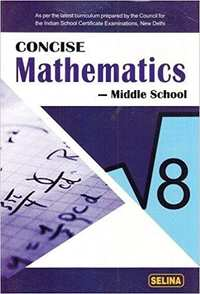## Chapter 17: Special Types of Quadrilaterals

Exercise 17
Exercise 17 [Pages 198 - 199]

### Selina solutions for Concise Mathematics Class 8 ICSE Chapter 17 Special Types of Quadrilaterals Exercise 17 [Pages 198 - 199]

Exercise 17 | Q 1 | Page 198

In parallelogram ABCD, ∠A = 3 times ∠B. Find all the angles of the parallelogram. In the same parallelogram, if AB = 5x – 7 and CD = 3x +1 ; find the length of CD.

Exercise 17 | Q 2 | Page 198

In parallelogram PQRS, ∠Q = (4x – 5)° and ∠S = (3x + 10)°. Calculate : ∠Q and ∠R.

Exercise 17 | Q 3 | Page 198

In rhombus ABCD;
(i) if ∠A = 74° ; find ∠B and ∠C.
(ii) if AD = 7.5 cm ; find BC and CD.

Exercise 17 | Q 4 | Page 198

In square PQRS :
(i) if PQ = 3x – 7 and QR = x + 3 ; find PS
(ii) if PR = 5x and QR = 9x – 8. Find QS

Exercise 17 | Q 5 | Page 198

ABCD is a rectangle, if ∠BPC = 124°
Calculate : (i) ∠BAP (ii) ∠ADP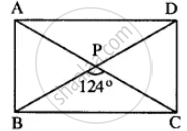Exercise 17 | Q 6 | Page 198

ABCD is a rhombus. If ∠BAC = 38°, find :
(i) ∠ACB
(ii) ∠DAC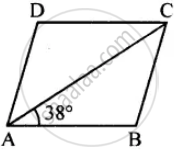Exercise 17 | Q 7 | Page 198

ABCD is a rhombus. If ∠BCA = 35°. find ∠ADC.

Exercise 17 | Q 8 | Page 198

PQRS is a parallelogram whose diagonals intersect at M.
If ∠PMS = 54°, ∠QSR = 25° and ∠SQR = 30° ; find :

(i) ∠RPS
(ii) ∠PRS
(iii) ∠PSR.

Exercise 17 | Q 9 | Page 198

Given: Parallelogram ABCD in which diagonals AC and BD intersect at M.
Prove: M is the mid-point of LN.

Exercise 17 | Q 10 | Page 198

In an Isosceles-trapezium, show that the opposite angles are supplementary.

Exercise 17 | Q 11.1 | Page 198

ABCD is a parallelogram. What kind of quadrilateral is it if : AC = BD and AC is perpendicular to BD?

Exercise 17 | Q 11.2 | Page 198

ABCD is a parallelogram. What kind of quadrilateral is it if: AC is perpendicular to BD but is not equal to it?

Exercise 17 | Q 11.3 | Page 198

ABCD is a parallelogram. What kind of quadrilateral is it if: AC = BD but AC is not perpendicular to BD?

Exercise 17 | Q 12 | Page 199

Prove that the diagonals of a parallelogram bisect each other.

Exercise 17 | Q 13 | Page 199

If the diagonals of a parallelogram are of equal lengths, the parallelogram is a rectangle. Prove it.

Exercise 17 | Q 14 | Page 199

In parallelogram ABCD, E is the mid-point of AD and F is the mid-point of BC. Prove that BFDE is a parallelogram.

Exercise 17 | Q 15 | Page 199

In parallelogram ABCD, E is the mid-point of side AB and CE bisects angle BCD. Prove that :

(ii) DE bisects and ∠ADC and
(iii) Angle DEC is a right angle.

Exercise 17 | Q 16 | Page 199

In the following diagram, the bisectors of interior angles of the parallelogram PQRS enclose a quadrilateral ABCD.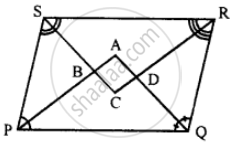Show that:
(i) ∠PSB + ∠SPB = 90°
(ii) ∠PBS = 90°
(iii) ∠ABC = 90°
(v) ∠A = 90°
(vi) ABCD is a rectangle
Thus, the bisectors of the angles of a parallelogram enclose a rectangle.

Exercise 17 | Q 17 | Page 199

In parallelogram ABCD, X and Y are midpoints of opposite sides AB and DC respectively. Prove that:

(i) AX = YC
(ii) AX is parallel to YC
(iii) AXCY is a parallelogram.

Exercise 17 | Q 18 | Page 199

The given figure shows parallelogram ABCD. Points M and N lie in diagonal BD such that DM = BN.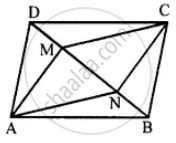Prove that:
(i) ∆DMC = ∆BNA and so CM = AN
(ii) ∆AMD = ∆CNB and so AM CN
(iii) ANCM is a parallelogram.

Exercise 17 | Q 19 | Page 199

The given figure shows a rhombus ABCD in which angle BCD = 80°. Find angles x and y.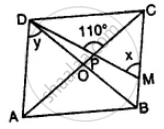Exercise 17 | Q 20 | Page 199

Use the information given in the alongside diagram to find the value of x, y, and z.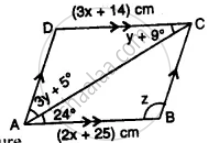Exercise 17 | Q 21 | Page 199

The following figure is a rectangle in which x: y = 3: 7; find the values of x and y.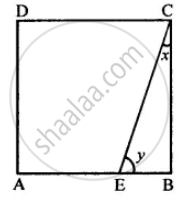Exercise 17 | Q 22 | Page 199

In the given figure, AB || EC, AB = AC and AE bisects ∠DAC. Prove that: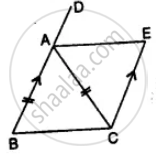(i) ∠EAC = ∠ACB
(ii) ABCE is a parallelogram.

## Chapter 17: Special Types of Quadrilaterals

Exercise 17## Selina solutions for Concise Mathematics Class 8 ICSE chapter 17 - Special Types of Quadrilaterals

Selina solutions for Concise Mathematics Class 8 ICSE chapter 17 (Special Types of Quadrilaterals) include all questions with solution and detail explanation. This will clear students doubts about any question and improve application skills while preparing for board exams. The detailed, step-by-step solutions will help you understand the concepts better and clear your confusions, if any. Shaalaa.com has the CISCE Concise Mathematics Class 8 ICSE solutions in a manner that help students grasp basic concepts better and faster.

Further, we at Shaalaa.com provide such solutions so that students can prepare for written exams. Selina textbook solutions can be a core help for self-study and acts as a perfect self-help guidance for students.

Concepts covered in Concise Mathematics Class 8 ICSE chapter 17 Special Types of Quadrilaterals are Properties of Trapezium, Properties of Rectangle, Properties of a Parallelogram, Properties of Rhombus, Property: The diagonals of a square are perpendicular bisectors of each other..

Using Selina Class 8 solutions Special Types of Quadrilaterals exercise by students are an easy way to prepare for the exams, as they involve solutions arranged chapter-wise also page wise. The questions involved in Selina Solutions are important questions that can be asked in the final exam. Maximum students of CISCE Class 8 prefer Selina Textbook Solutions to score more in exam.

Get the free view of chapter 17 Special Types of Quadrilaterals Class 8 extra questions for Concise Mathematics Class 8 ICSE and can use Shaalaa.com to keep it handy for your exam preparation